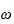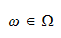StatLect

# Sample point

In a probabilistic experiment, a sample point is one of the possible outcomes of the experiment. The set of all sample points is called sample space.## Notation

The sample space is usually denoted by the Greek letter(Omega) and a sample point is indicated by its lowercase version(omega):## Example

Suppose the random experiment is the flip of a coin. It can give rise to two possible outcomes: tail () and head ().andare the two sample points andis the sample space.

## More details

The notions of sample space and sample point are discussed in detail in the lecture entitled Probability.

Previous entry: Sample mean

Next entry: Sample size